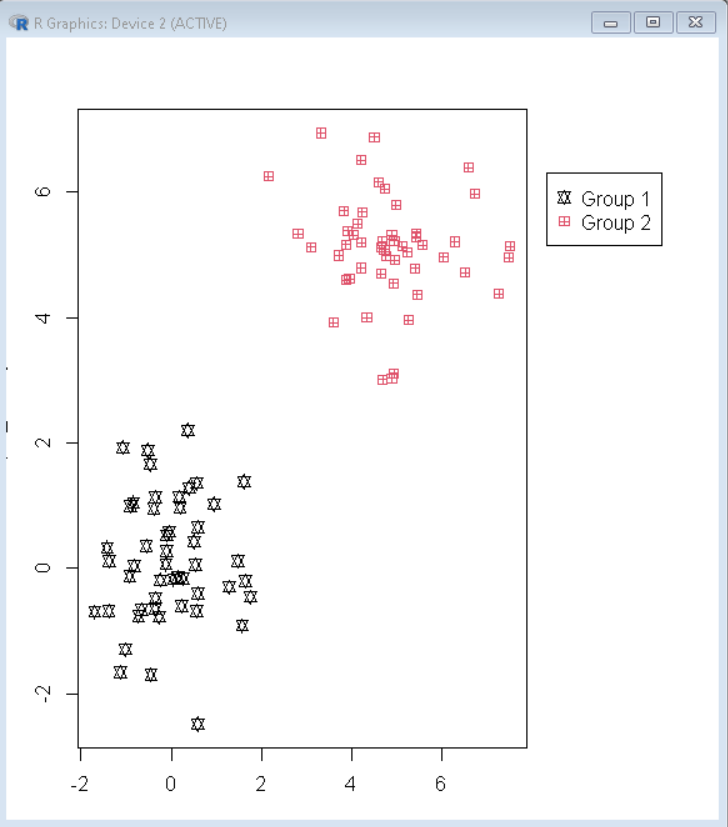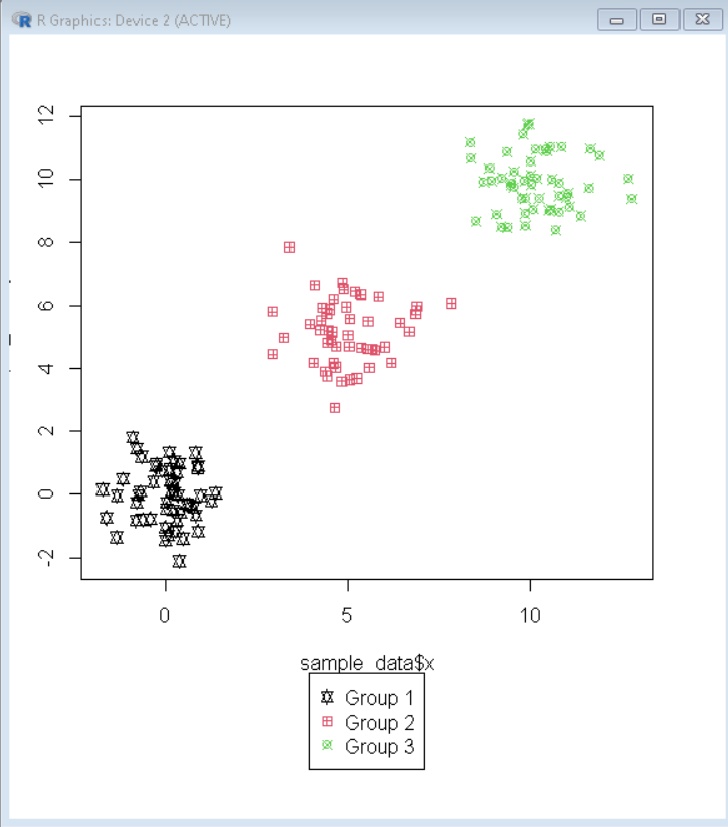# How to Draw a Legend Outside of a Plot in R?

• Last Updated : 19 Dec, 2021

In this article, we will discuss how to draw a legend outside of a Plot in the R Programming Language.

We first create a basic plot without legend using the plot() function and add a margin around it for legend by using the par() function of the R Language. We will create the required margin and make the xpd parameter TRUE. This will make our plotting clipped to the figure region.

Syntax:

`par( mar, xpd)`

where,

• mar: determines the vector which contains margin.
• xpd: It is a boolean. If FALSE, all plotting is clipped to the plot region, if TRUE, all plotting is clipped to the figure region

Then we use the legend() function to add a legend layer on top of it. To put the legend at the desired position on the plot we use the inset parameter of the legend function.

Syntax:

`legend(position, inset, title, legend, pch, col )`

where,

• position: determines the position of the legend.
• inset: determines the shift in position.
• title: determines the title of legend.
• pch: determines the symbols used to represent the data point.
• col: determines the color of data points.

Example 1:

Here, is a basic plot in the R Language with the legend on the top right corner of the plot.

## R

 `# create sample data frame``sample_data <- ``data.frame``(x = ``c``(``rnorm``(50), ``rnorm``(50, 5)),      ``                   ``y = ``c``(``rnorm``(50), ``rnorm``(50, 5)),``                   ``group = ``c``(``rep``(1, 50), ``rep``(2, 50))) `` ` `# create margin around plot``par``(mar = ``c``(3, 3, 3, 8), xpd = ``TRUE``)`` ` `# Draw scatter plot``plot``(sample_data\$x, sample_data\$y,                                   ``     ``pch = sample_data\$group+10,``     ``col = sample_data\$group)`` ` `# Draw legend``legend``(``"topright"``, inset = ``c``(-0.3, 0.1),                   ``       ``legend = ``c``(``"Group 1"``,``"Group 2"``),``       ``pch = ``c``(11,12), col = 1:2)`

Output:Example 2:

Here, is a basic plot in the R Language with the legend on the bottom of the plot.

## R

 `# create sample data frame``sample_data <- ``data.frame``(x = ``c``(``rnorm``(50), ``rnorm``(50, 5), ``rnorm``(50, 10)),      ``                   ``y = ``c``(``rnorm``(50), ``rnorm``(50, 5), ``rnorm``(50, 10)),``                   ``group = ``c``(``rep``(1, 50), ``rep``(2, 50), ``rep``(3, 50))) `` ` `# create margin around plot``par``(mar = ``c``(10, 3, 3, 3), xpd = ``TRUE``)`` ` `# Draw scatter plot``plot``(sample_data\$x, sample_data\$y,                                   ``     ``pch = sample_data\$group+10,``     ``col = sample_data\$group)`` ` `# Draw legend``legend``(``"topright"``, inset = ``c``(0.4, 1.2),                   ``       ``legend = ``c``(``"Group 1"``,``"Group 2"``,``"Group 3"``),``       ``pch = ``c``(11,12,13), col = 1:3)`

Output:My Personal Notes arrow_drop_up